## Spheroid

A spheroid is an Ellipsoid(1)

with two Semimajor Axes equal. Orient the Ellipse so that theandaxes are equal, then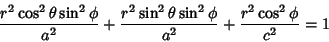(2)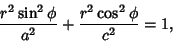(3)

whereis the equatorial Radius and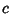is the polar Radius. Here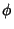is the colatitude, so take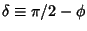to express in terms of latitude.(4)

Rewritinggives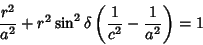(5)(6)

so(7)

If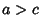, the spheroid is Oblate. If, the spheroid is Prolate. If, the spheroid degenerates to a Sphere.